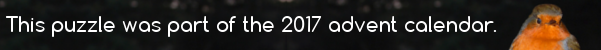mscroggs.co.uk
mscroggs.co.uksubscribe

# Puzzles

## 6 December$$p(x)$$ is a quadratic with real coefficients. For all real numbers $$x$$,
$$x^2+4x+14\leq p(x)\leq 2x^2+8x+18$$
$$p(2)=34$$. What is $$p(6)$$?

Source: Luciano Rila (@DrTrapezio)
$$p(x)$$ is a quadratic polynomial with real coefficients. For all real numbers $$x$$,
$$x^2-2x+2\leq p(x)\leq 2x^2-4x+3$$
$$p(11)=181$$. Find $$p(16)$$.

## Balanced sets

A set of points in the plane is called 'balanced' if for any two points $$A$$ and $$B$$ in the set, there is another point $$C$$ in the set such that $$AC=BC$$ (here $$AC$$ is the distance between $$A$$ and $$C$$).
For all $$n\geq3$$, find a balanced set of $$n$$ points.

## Bézier curve

A Bézier curve is created as follows:
1) A set of points $$P_0$$, ..., $$P_n$$ are chosen (in the example $$n=4$$).
2) A set of points $$Q_0$$, ..., $$Q_{n-1}$$ are defined by $$Q_i=t P_{i+1}+(1-t) P_i$$ (shown in green).
3) A set of points $$R_0$$, ..., $$R_{n-2}$$ are defined by $$R_i=t Q_{i+1}+(1-t) Q_i$$ (shown in blue).
.
.
.
$$n$$) After repeating the process $$n$$ times, there will be one point. The Bézier curve is the path traced by this point at $$t$$ varies between 0 and 1.

What is the Cartesian equation of the curve formed when:
$$P_0=\left(0,1\right)$$ $$P_1=\left(0,0\right)$$ $$P_2=\left(1,0\right)$$

## Parabola

On a graph of $$y=x^2$$, two lines are drawn at $$x=a$$ and $$x=-b$$ (for $$a,b>0$$. The points where these lines intersect the parabola are connected.
What is the y-coordinate of the point where this line intersects the y-axis?

## Two lines

Let A and B be two straight lines such that the gradient of A is the y-intercept of B and the y-intercept of A is the gradient of B (the gradient and y-intercept of A are not the same). What are the co-ordinates of the point where the lines meet?

## Archive

Show me a random puzzle
▼ show ▼